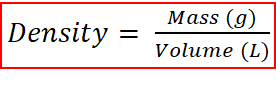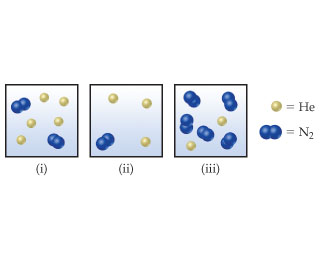# Problem: Consider the following samples of gases:If the three samples are all at the same temperature, rank them with respect to density (d).

###### FREE Expert Solution

Density establishes a relationship between mass and volumewhere Mass is in grams and Volume in L.

Note, the volume of all the three samples are the same as they are all in boxes of equal dimensions. Therefore, the mass of each sample has to be compared in order to understand the difference in the density of each sample.

87% (436 ratings)###### Problem Details

Consider the following samples of gases:If the three samples are all at the same temperature, rank them with respect to density (d).